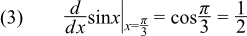#Boost C++ Libraries

...one of the most highly regarded and expertly designed C++ library projects in the world.

##### Calculating a Derivative

In this example we'll add even more power to generic numeric programming using not only different floating-point types but also function objects as template parameters. Consider some well-known central difference rules for numerically computing the first derivative of a function f′(x) with x ∈ ℜ:Where the difference terms mn are given by:and dx is the step-size of the derivative.

The third formula in Equation 1 is a three-point central difference rule. It calculates the first derivative of f′(x) to O(dx6), where dx is the given step-size. For example, if the step-size is 0.01 this derivative calculation has about 6 decimal digits of precision - just about right for the 7 decimal digits of single-precision float. Let's make a generic template subroutine using this three-point central difference rule. In particular:

```template<typename value_type, typename function_type>
value_type derivative(const value_type x, const value_type dx, function_type func)
{
// Compute d/dx[func(*first)] using a three-point
// central difference rule of O(dx^6).

const value_type dx1 = dx;
const value_type dx2 = dx1 * 2;
const value_type dx3 = dx1 * 3;

const value_type m1 = (func(x + dx1) - func(x - dx1)) / 2;
const value_type m2 = (func(x + dx2) - func(x - dx2)) / 4;
const value_type m3 = (func(x + dx3) - func(x - dx3)) / 6;

const value_type fifteen_m1 = 15 * m1;
const value_type six_m2     =  6 * m2;
const value_type ten_dx1    = 10 * dx1;

return ((fifteen_m1 - six_m2) + m3) / ten_dx1;
}
```

The `derivative()` template function can be used to compute the first derivative of any function to O(dx6). For example, consider the first derivative of sin(x) evaluated at x = π/3. In other words,The code below computes the derivative in Equation 3 for float, double and boost's multiple-precision type cpp_dec_float_50.

```#include <iostream>
#include <iomanip>
#include <boost/multiprecision/cpp_dec_float.hpp>
#include <boost/math/constants/constants.hpp>

int main(int, char**)
{
using boost::math::constants::pi;
using boost::multiprecision::cpp_dec_float_50;
//
// We'll pass a function pointer for the function object passed to derivative,
// the typecast is needed to select the correct overload of std::sin:
//
const float d_f = derivative(
pi<float>() / 3,
0.01F,
static_cast<float(*)(float)>(std::sin)
);

const double d_d = derivative(
pi<double>() / 3,
0.001,
static_cast<double(*)(double)>(std::sin)
);
//
// In the cpp_dec_float_50 case, the sin function is multiply overloaded
// to handle expression templates etc.  As a result it's hard to take its
// C++11 lambda expression to capture the call.
// We also need a typecast on the first argument so we don't accidentally pass
// an expression template to a template function:
//
const cpp_dec_float_50 d_mp = derivative(
cpp_dec_float_50(pi<cpp_dec_float_50>() / 3),
cpp_dec_float_50(1.0E-9),
[](const cpp_dec_float_50& x) -> cpp_dec_float_50
{
return sin(x);
}
);

// 5.000029e-001
std::cout
<< std::setprecision(std::numeric_limits<float>::digits10)
<< d_f
<< std::endl;

// 4.999999999998876e-001
std::cout
<< std::setprecision(std::numeric_limits<double>::digits10)
<< d_d
<< std::endl;

// 4.99999999999999999999999999999999999999999999999999e-01
std::cout
<< std::setprecision(std::numeric_limits<cpp_dec_float_50>::digits10)
<< d_mp
<< std::endl;
}
```

The expected value of the derivative is 0.5. This central difference rule in this example is ill-conditioned, meaning it suffers from slight loss of precision. With that in mind, the results agree with the expected value of 0.5.

We can take this a step further and use our derivative function to compute a partial derivative. For example if we take the incomplete gamma function P(a, z), and take the derivative with respect to z at (2,2) then we can calculate the result as shown below, for good measure we'll compare with the "correct" result obtained from a call to gamma_p_derivative, the results agree to approximately 44 digits:

```cpp_dec_float_50 gd = derivative(
cpp_dec_float_50(2),
cpp_dec_float_50(1.0E-9),
[](const cpp_dec_float_50& x) ->cpp_dec_float_50
{
return boost::math::gamma_p(2, x);
}
);
// 2.70670566473225383787998989944968806815263091819151e-01
std::cout
<< std::setprecision(std::numeric_limits<cpp_dec_float_50>::digits10)
<< gd
<< std::endl;
// 2.70670566473225383787998989944968806815253190143120e-01
std::cout << boost::math::gamma_p_derivative(cpp_dec_float_50(2), cpp_dec_float_50(2)) << std::endl;
```